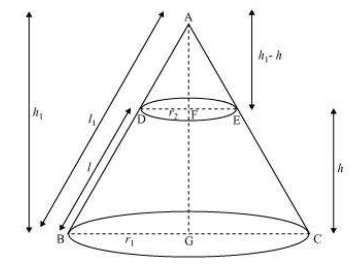# Derive the formula for the curved surface area and total surface area of the frustum of a cone, given to you in Section 13.5, using the symbols as explained.Let us assume

ABC is a cone.

From the cone, the frustum DECB is cut by a plane parallel to its base.

Here, r1 and r2 are the radii of the frustum ends of the cone and h be the frustum height.

Let us consider the ΔABG and ΔADF,

Here, DF||BG

$$\frac{DF}{BG}=\frac{AF}{AG}=\frac{AD}{AB}$$

\frac{h_{1}-h}{h_{1}}

On rearranging the terms, we get

$$l^{1}=\frac{r_{1}l}{r_{1}-r_{2}}$$

The total surface area of the frustum =total CSA of frustum + the area of upper circular end + area of the lower circular end

= π(r1+r2)l+πr22+πr12

∴ Surface area of frustum = π[r1+r2)l+r12+r22]